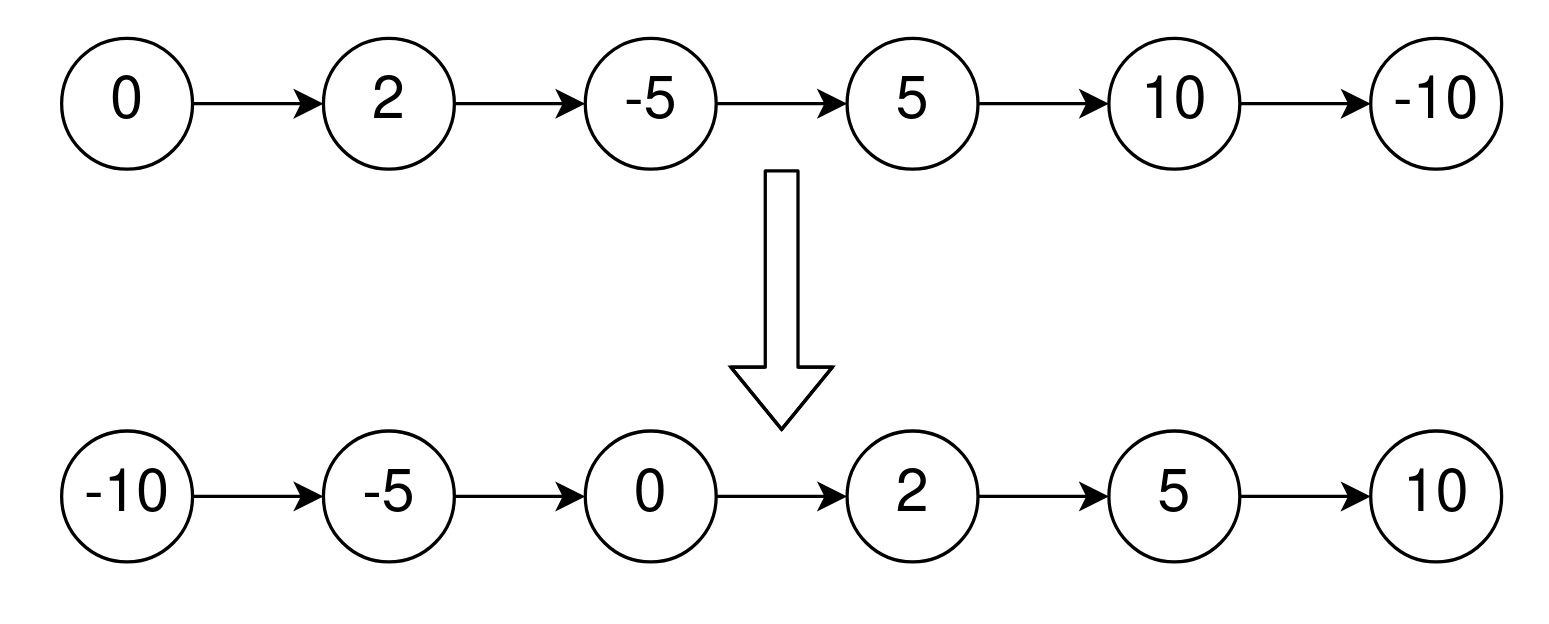##### Welcome to Subscribe On Youtube

Formatted question description: https://leetcode.ca/all/2046.html

Given the head of a singly linked list that is sorted in non-decreasing order using the absolute values of its nodes, return the list sorted in non-decreasing order using the actual values of its nodes.

Example 1:Input: head = [0,2,-5,5,10,-10]
Output: [-10,-5,0,2,5,10]
Explanation:
The list sorted in non-descending order using the absolute values of the nodes is [0,2,-5,5,10,-10].
The list sorted in non-descending order using the actual values is [-10,-5,0,2,5,10].


Example 2:Input: head = [0,1,2]
Output: [0,1,2]
Explanation:


Example 3:

Input: head = 
Output: 
Explanation:


Constraints:

• The number of nodes in the list is the range [1, 105].
• -5000 <= Node.val <= 5000
• head is sorted in non-decreasing order using the absolute value of its nodes.

• Can you think of a solution with O(n) time complexity?

Related Topics:

Similar Questions:

## Solution 1.

• /**
* public class ListNode {
*     int val;
*     ListNode next;
*     ListNode() {}
*     ListNode(int val) { this.val = val; }
*     ListNode(int val, ListNode next) { this.val = val; this.next = next; }
* }
*/
class Solution {
while (curr != null) {
if (curr.val < 0) {
ListNode t = curr.next;
prev.next = t;
curr = t;
} else {
prev = curr;
curr = curr.next;
}
}
}
}

• /**
* struct ListNode {
*     int val;
*     ListNode *next;
*     ListNode() : val(0), next(nullptr) {}
*     ListNode(int x) : val(x), next(nullptr) {}
*     ListNode(int x, ListNode *next) : val(x), next(next) {}
* };
*/
class Solution {
public:
while (curr) {
if (curr->val < 0) {
auto t = curr->next;
prev->next = t;
curr = t;
} else {
prev = curr;
curr = curr->next;
}
}
}
};

• # Definition for singly-linked list.
# class ListNode:
#     def __init__(self, val=0, next=None):
#         self.val = val
#         self.next = next
class Solution:
while curr:
if curr.val < 0:
t = curr.next
prev.next = t
curr = t
else:
prev, curr = curr, curr.next


• /**
* type ListNode struct {
*     Val int
*     Next *ListNode
* }
*/
for curr != nil {
if curr.Val < 0 {
t := curr.Next
prev.Next = t